# Number and its Types in Julia

Julia is a high-level, dynamic, general-purpose programming language that can be used to write software applications, and is well-suited for data analysis and computational science. Numbers are important in any programming language. Numbers in Julia is classified into two types: Integer and Floating-Point Numbers. Julia provides a wide range of primitive numeric types. These inbuilt numeric datatypes help Julia to take full advantage of computational resources. Julia also provides software support for Arbitrary Precision Arithmetic, which can handle numeric operations which is difficult to represent in native hardware representations. However, support for Arbitrary Precision Arithmetic comes at the cost of slower performance. Complex and Rational Numbers are defined on top of primitive numeric types.

The following are Julia’s primitive numeric types:

1. Integer
Type Signed Number of bits Range
Int8 Yes 8 -2^7 to 2^7 – 1
UInt8 No 8 0 to 2^8-1
Int16 Yes 16 -2^15 to 2^15-1
UInt16 No 16 0 to 2^16 – 1
Int32 Yes 32 -2^31 to 2^31-1
UInt32 No 32 0 to 2^32-1
Int64 Yes 64 -2^63 to 2^63-1
UInt64 No 64 0 to 2^64 – 1
Int128 Yes 128 -2^127 to 2^127 – 1
UInt128 No 128 0 to 2^128 – 1
Bool N/A 8 false(0) and true(1)
2. Floating point numbers
Type Precision Number of bits
Float16 half 16
Float32 single 32
Float64 double 64

#### Integers

The default type for an integer literal depends on whether the system is 32-bit or 64-bit. The variable Sys.WORD_SIZE indicates whether the system is 32-bit or 64-bit:

 `println(Sys.WORD_SIZE) ` `println(typeof(``123``)) `

Output: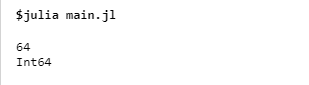However, larger integer literals that cannot be represented using 32 bits are represented using 64 bits, regardless of the system type.
Unsigned integers are represented using the 0x prefix and hexadecimal digits 0-9a-f or 0-9A-F. Size of the unsigned integer is determined by the number of hex digits used.

 `println(typeof(``0x12``)) ` `println(typeof(``0x123``)) ` `println(typeof(``0x1234567``)) ` `println(typeof(``0x123456789abcd``)) ` `println(typeof(``0x1112223333444555566677788``)) `

Output: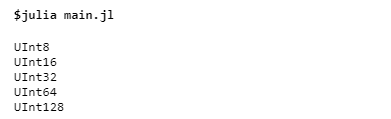Binary and octal literals are also supported in Julia.

 `println(typeof(``0b10``)) ` `println(typeof(``0o10``)) ` `println(``0b10``) ` `println(``0o10``) `

Output: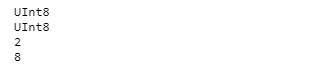##### Min-Max Values of Integers

 `println(typemin(Int8), ``' '``, typemax(Int8)) ` `println(typemin(Int16), ``' '``, typemax(Int16)) ` `println(typemin(Int32), ``' '``, typemax(Int32)) ` `println(typemin(Int64), ``' '``, typemax(Int64)) ` `println(typemin(Int128), ``' '``, typemax(Int128)) `

Output: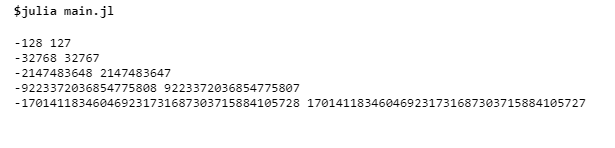#### Floating Point Numbers

Floating Point Numbers are represented in a standard format. There is no literal format for Float32, but we can convert values to Float32 by writing an ‘f’ or explicit typecasting.

 `println(typeof(``1.0``)) ` `println(typeof(.``5``)) ` `println(typeof(``-``1.23``)) ` `println(typeof(``0.5f0``)) ` `println(``2.5f``-``4``) ` `println(typeof(``2.5f``-``4``)) ` `println(typeof(Float32(``-``1.5``))) `

Output: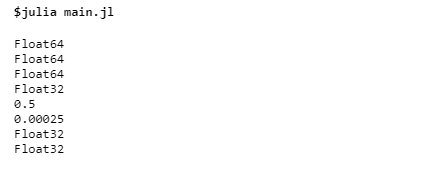##### Floating point zero

Floating point numbers have a positive zero and a negative zero which are equal to each other but have different binary representations.

```julia> 0.0 == -0.0
true

julia> bitstring(0.0)
"0000000000000000000000000000000000000000000000000000000000000000"

julia> bitstring(-0.0)
"1000000000000000000000000000000000000000000000000000000000000000"
```
##### Special floating-point values
Name Type Description
positive infinity Inf16, Inf32, Inf a value greater than all finite floating-point values
negative infinity -Inf16, -Inf32, -Inf a value less than all finite floating-point values
not a number NaN16, NaN32, NaN a value not comparable to any floating point values including itself

#### Complex Number

Global constant ‘im’ is used to represent complex number i where i is square root of -1.

 `println(typeof(``1``+``2im``)) ` ` `  `# performing mathematical operations ` ` `  `println() ` `println((``1` `+` `2im``) ``+` `(``2` `+` `3im``)) ` `println((``5` `+` `5im``) ``-` `(``3` `+` `2im``)) ` `println((``5` `+` `2im``) ``*` `(``3` `+` `2im``)) ` `println((``1` `+` `2im``) ``/` `(``1` `-` `2im``)) ` `println(``3``(``2` `-` `5im``)^``2``) `

Output: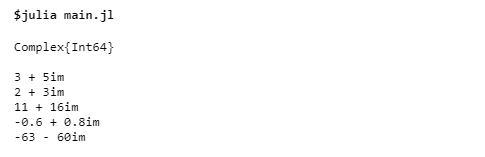##### Rational Number

Julia has rational numbers to represent exact ratios of integers. Rational numbers are constructed using the // operator.

 `println(typeof(``6``/``/``9``)) ` `println(``6``/``/``9``) ` `println(``-``6``/``/``9``) ` `println(``-``6``/``/``-``9``) ` `println(``5``/``/``8` `+` `3``/``/``12``) ` `println(``6``/``/``5` `-` `10``/``/``13``) ` `println(``5``/``/``8` `*` `3``/``/``12``) ` `println(``6``/``/``5` `/` `10``/``/``3``) `

Output: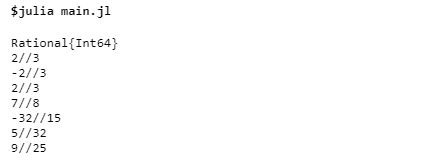My Personal Notes arrow_drop_upIf you like GeeksforGeeks and would like to contribute, you can also write an article using contribute.geeksforgeeks.org or mail your article to contribute@geeksforgeeks.org. See your article appearing on the GeeksforGeeks main page and help other Geeks.

Please Improve this article if you find anything incorrect by clicking on the "Improve Article" button below.

Article Tags :

Be the First to upvote.

Please write to us at contribute@geeksforgeeks.org to report any issue with the above content.